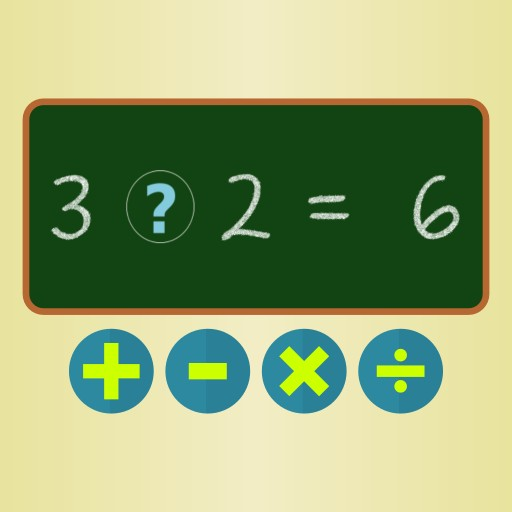## Sinal Game## Sinal Game

Description:

In this mathematical game the player must observe the mathematical equation and answer which is the correct operator of the equations (addition, subtraction, division and multiplication). The goal is to reach the highest score possible in 60 seconds. Hits add 2 to the score, but misses decrease it by 1.

Instructions:

Mouse or touch to select the operators.

Favorite:
Play it online!
Game not working?
×
Sinal Game is not working?
* You should use least 20 words.

## Sinal Game Video Walkthrough

Description:

In this mathematical game the player must observe the mathematical equation and answer which is the correct operator of the equations (addition, subtraction, division and multiplication). The goal is to reach the highest score possible in 60 seconds. Hits add 2 to the score, but misses decrease it by 1.

Instructions:

Mouse or touch to select the operators.